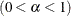### Autocall Macros for Postprocessing

Although PROC MCMC provides a number of convergence diagnostic tests and posterior summary statistics, PROC MCMC performs the calculations only if you specify the options in advance. If you wish to analyze the posterior draws of unmonitored parameters or functions of the parameters that are calculated in later DATA step calls, you can use the autocall macros in Table 55.44.

Table 55.44: Postprocessing Autocall Macros

Macro

Description

%ESS

Effective sample sizes

%GEWEKE[a]

Geweke diagnostic

%HEIDELfnMacroOpt

Heidelberger-Welch diagnostic

%MCSE

Monte Carlo standard errors

%RAFTERY

Raftery diagnostic

%POSTACF

Autocorrelation

%POSTCOR

Correlation matrix

%POSTCOV

Covariance matrix

%POSTINT

Equal-tail and HPD intervals

%POSTSUM

Summary statistics

[a] The %GEWEKE and %HEIDEL macros use a different optimization routine than that used in PROC MCMC. As a result, there might be numerical differences in some cases, especially when the sample size is small.

Table 55.45 lists options that are shared by all postprocessing autocall macros. See Table 55.46 for macro-specific options.

Table 55.45: Shared Options

Option

Description

DATA=SAS-data-set

Input data set that contains posterior samples

VAR=variable-list

Specifies the variables on which you want to carry out the calculation.

PRINT=YES | NO

Displays the results. The default is YES.

OUT=SAS-data-set

Specifies a name for the output SAS data set to contain the results.

Suppose that the data set that contains posterior samples is called post and that the variables of interest are defined in the macro variable &PARMS. The following statements call the %ESS macro and calculates the effective sample sizes for each variable:

%let parms = alpha beta u_1-u_17;
%ESS(data=post, var=&parms);


By default, the ESS estimates are displayed. You can choose not to display the result and save the output to a data set with the following statement:

%ESS(data=post, var=&parms, print=NO, out=eout);


Some of the macros can take additional options, which are listed in Table 55.46.

Table 55.46: Macro-Specific Options

Macro

Option

Description

%ESS

AUTOCORLAG=numeric

Specifies the maximum number of autocorrelation lags used in computing the ESS estimates. By default, AUTOCORLAG=MIN(500, NOBS/4), where NOBS is the sample size of the input data set.

HIST=YES|NO

Displays a histogram of all ESS estimates. The default is NO.

%HEIDEL

SALPHA=numeric

Specifies thelevel for the stationarity test. By default, SALPHA=0.05.

HALPHA=numeric

Specifies thelevel for the halfwidth test. By default, HALPHA=0.05.

EPS=numeric

Specifies a small positive numbersuch that if the halfwidth is less thantimes the sample mean of the remaining iterations, the halfwidth test is passed. By default, EPS=0.1.

%GEWEKE

FRAC1=numeric

Specifies the earlier portion of the Markov chain used in the test. By default, FRAC1=0.1.

FRAC2=numeric

Specifies the latter portion of the Markov chain used in the test. By default, FRAC2=0.5.

%MCSE

AUTOCORLAG=numeric

Specifies the maximum number of autocorrelation lags used in computing the Monte Carlo standard error estimates. By default, AUTOCORLAG=MIN(500, NOBS/4), where NOBS is the sample size of the input data set.

%RAFTERY

Q=numeric

Specifies the order of the quantile of interest. By default, Q=0.025.

R=numeric

Specifies the margin of error for measuring the accuracy of estimation of the quantile. By default, R=0.005.

S=numeric

Specifies the probability of attaining the accuracy of the estimation of the quantile. By default, S=0.95.

EPS=numeric

Specifies the tolerance level for the stationary test. By default, EPS=0.001.

%POSTACF

LAGS=%str(numeric-list)

Specifies autocorrelation lags calculated. The default values are 1, 5, 10, and 50.

%POSTINT

ALPHA=value

Specifies thelevelfor the interval estimates. By default, ALPHA=0.05.

For example, the following statement calculates and displays autocorrelation at lags 1, 6, 11, 50, and 100. Note that the lags in the numeric-list need to be separated by commas “,”.

%PostACF(data=post, var=&parms, lags=%str(1 to 15 by 5, 50, 100));